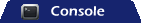# Java Collections – BitSet Example

The BitSet implements a vector of bits are can dynamically expand as needed. With this structure, each element, or bit represents a boolean value of true (set) or false (unset). We can set, clear and return any value of the BitSet using the set(), clear() and get() methods respectively. Additionally, we can modify one BitSet by using another BitSet and applying the logical operators of (and(), andnot(), or() and xor()).

### Worthy Note

Please note that the size of a BitSet will be 64 even if you only instantiate a BitSet with initialSize of 2 or any capacity in the range of (0 ~ 64);

```BitSet set = new BitSet(2);
System.out.println(set.size()); // should be 64
BitSet set1 = new BitSet(23);
System.out.println(set1.size()); // should be 64
BitSet set2 = new BitSet(69);
System.out.println(set2.size()); // should be 128
```

### Creating a BitSet

`BitSet questions = new BitSet();`

or

`BitSet questions = new BitSet(int initialSize);`

### Setting on Bits

Setting bits to true on the questions BitSet is done by using the set(int index) method.

```questions.set(1);
questions.set(2);
// Set bits 5 through 7 to true
questions.set(5, 8);
```

### Clearing Bits

Clearing any bit is just a matter of calling the method clear(int index).

```// Clears the bit at #5 (sets it to false)
System.out.print("Clearing bit #5: ");
questions.clear(5);
```

you can also clear a bit by using the set(int index, boolean value). Actually, this method could be used to set both true/false values.

```questions.set(2, true);
questions.set(4, false);
questions.set(8, true);
```

### Flipping Bits

Flipping any bit is just a matter of calling the method flip(int index).

```Flipping bit at #4 True->False, False->True
System.out.print("Flipping bit #4: ");
questions.flip(4);
```

you can also flip a range of bits by using the flip(int fromIndex, int toIndex).

```// Flipping bit at #6 ~ 10
questions.flip(6, 10);```

### Size of Collection

*NOTE: Here is where BitSet differs from the other structures in the collections framework. It actually returns the number of bits of space in use by this BitSet to represent bit values. Use the size() method to see how much space your collection is actually taking up.

`questions.size();`

### Iterating through the Collection

```System.out.println("nLooking at the entire BitSet...");
// Display the collection of bits in questions
for (int i = 0; i < MAX_QUESTIONS; i++) {
System.out.println(i + ": " + questions.get(i));
}
```

### Full Program Listing (BitSetExample.java)

```package com.avaldes.tutorials;

import java.util.BitSet;

public class BitSetExample {

public static void main(String[] args) {
final int MAX_QUESTIONS = 10;
BitSet questions = new BitSet(MAX_QUESTIONS);

System.out.print("BitSet Example: ");
System.out.println(questions);

// Set the bit at #2 to true
System.out.print("Setting bit #2: ");
questions.set(2);
System.out.println(questions);

// Set bits 5 through 7 to true
System.out.print("Setting bits #5 ~ #7: ");
questions.set(5, 8);
System.out.println(questions);

// Clears the bit at #5 (sets it to false)
System.out.print("Clearing bit #5: ");
questions.clear(5);
System.out.println(questions);

// Unset bit at #2 to false -- not the preferred way to do it.
System.out.print("Clearing bit #2: ");
questions.set(2, false);
System.out.println(questions);

// Flipping bit at #4 True->False, False->True
System.out.print("Flipping bit #4: ");
questions.flip(4);
System.out.println(questions);

// Flipping bit at #6 ~ 10
System.out.print("Flipping bits #6 ~ #9: ");
questions.flip(6, 10);
System.out.println(questions);

System.out.println("nLooking at the entire BitSet...");
// Display the collection of bits in questions
for (int i = 0; i < MAX_QUESTIONS; i++) {
System.out.println(i + ": " + questions.get(i));
}
}
}
```

### Output```BitSet Example: {}
Setting bit #2: {2}
Setting bits #5 ~ #7: {2, 5, 6, 7}
Clearing bit #5: {2, 6, 7}
Clearing bit #2: {6, 7}
Flipping bit #4: {4, 6, 7}
Flipping bits #6 ~ #9: {4, 8, 9}

Looking at the entire BitSet...
0: false
1: false
2: false
3: false
4: true
5: false
6: false
7: false
8: true
9: true
```